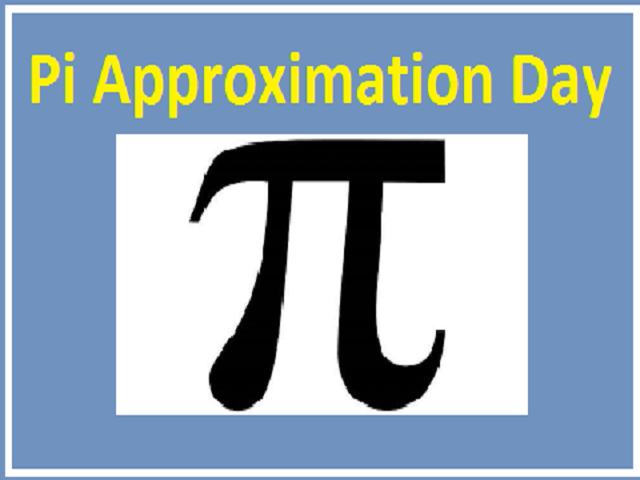# Pi Approximation Day 2021: Date, History, Significance, Quotes, Facts and more

Pi Approximation Day 2021: It is observed on 22 July and is also known as Casual Pi Day. The day is dedicated to the mathematical constant pi (π). Let us read more about the day.
Created On: Jul 22, 2021 06:40 IST
Modified On: Jul 22, 2021 06:44 ISTPi Approximation Day

Pi Approximation Day 2021: The ratio of the circumference of any circle to the diameter of that circle is Pi. In the decimal form, the value of pi (π) is 3.14. π symbol was devised by British mathematician William Jones in 1706 to represent the ratio and later it was popularised by the Swiss mathematician Leonhard Euler.

22 July is observed as Pi Approximation Day and 14 March as Pi Day. Pi Approximation Day is also known as Casual Pi Day.

Pi Approximation Day depends upon the format of the Date

According to timeanddate.com, the day depends upon the format of the Date. In some countries where people write their dates in the date/month format celebrate Pi Approximation Day or Casual Pi Day on 22 July or 22/7. And on the other hand, those who write their date in month/date format celebrate Pi Day on 14 March (3/14 or 3-14). It is because the first three digits of the date corresponding to the first three digits of pi (3.14).

- pi is irrational that is not equal to the ratio of any two whole numbers. Its digits do not repeat and for everyday calculations, an approximation like 3.14 or 22/7 is often used.

- The value of pi to 39 decimal places is 3.141592653589793238462643383279502884197.

- The Babylonians used 3,125 to approximate pi which is a value they obtained by calculating the perimeter of a hexagon inscribed within a circle and assume the ratio of the hexagon's perimeter to the circumference of the circle and it was 24/25.

- It's indicated by the Rhind papyrus that ancient Egyptians used a value of 256/81 or about 3.16045.

- A major step was taken forward by the Archimedes of devising a method to obtain pi to any desired accuracy. It was done by inscribing and circumscribing regular polygons about a circle to obtain upper and lower bounds and he obtained 223/71 < π < 22/7, or an average value of about 3.1418.

- It was also proved by Archimedes that the ratio of the area of a circle to the square of its radius is the same constant.

­- By the end of the 17th century, new methods of mathematical analysis in Europe provided improved ways of calculating pi that involves infinite series. For example, Sir Issac Newton used his binomial theorem to calculate 16 decimal places quickly.

­ - The Indian mathematician Srinivasa Ramanujan early in the 20th century developed exceptionally efficient ways of calculating pi and was later incorporated into computer algorithms.

- Computers calculated pi to 31,415,926,535,897 decimal places in the early 21st century and also its two-quadrillionth digit when expressed in binary (0).

- Pi is used in several mathematical problems consisting of the lengths of arcs or other surfaces like the areas of ellipses, sectors, and other curved surfaces, and the volumes of various solids.

- Pi is also used in several formulas of physics and engineering to describe such periodic phenomena as the motion of pendulums, the vibrations of strings, and alternating electric currents.

Pi Approximation Day: Quotes

1. "We cannot hope that many children will learn mathematics unless we find a way to share our enjoyment and show them its beauty as well as its utility." - Mary Beth

2. "It’s exact and indefinite. It’s like pi- you can keep figuring it out and always be right and never be done." -  Unknown

3. "Pi is not merely the ubiquitous factor in high school geometry problems; it is stitched across the whole tapestry of mathematics, not just geometry’s little corner of it." -  Unknown

4. “Errors using inadequate data are much less than those using no data at all.” - Charles Babbage

5. “Mathematicians are like managers – they want improvement without change.” - Edsger Dijkstra

6. “Mathematicians stand on each other’s shoulders.” - Carl Friedrich Gauss

7. “What is imagination?…It is a God-like, a noble faculty. It renders earth tolerable, it teaches us to live, in the tone of the eternal.”- Ada Lovelace

8. "The Great Pyramid, that monument to spirituality that the Agashan Teachers hold in such high esteem, is built according to Pi’s principles." - William Eisen

9. "Exploring pi is like exploring the universe." - David Chudnovsky

10. "Pi is not merely the ubiquitous factor in high school geometry problems; it is stitched across the whole tapestry of mathematics, not just geometry’s little corner of it." - Robert Kanigel

Important Days and Dates in July 2021

## FAQ

### Who devised the symbol π?

π symbol was devised by British mathematician William Jones in 1706 to represent the ratio and later it was popularised by the Swiss mathematician Leonhard Euler.

### What is the value of pi to 39 decimal places?

The value of pi to 39 decimal places is 3.141592653589793238462643383279502884197.

### What is Pi Approximation Day observed?

Pi Approximation Day is observed on 22 July and is also known as Casual Pi Day. The day is dedicated to the mathematical constant pi (π).
Comment ()

6 + 8 =
Post